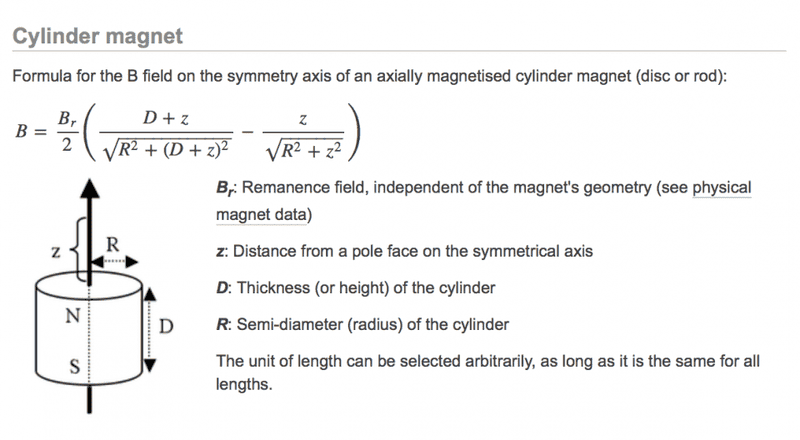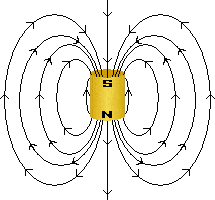# Flux Density, B, of a Cylindrical Magent

Bri1
Hi, I'm trying to calculate the flux density of a magnet, I can get all but one of the values needed to calculate it. Does anyone know how/where to get the z(distance from a pole face on the symmetrical axis) value?Mentor
Hi, I'm trying to calculate the flux density of a magnet, I can get all but one of the values needed to calculate it. Does anyone know how/where to get the z(distance from a pole face on the symmetrical axis) value?View attachment 113520
Welcome to the PF.

z is just a variable. It looks like you have an equation there which would work along the z axis for varying distances, no?

BTW, is this for schoolwork or a hobby project?

Bri1
Welcome to the PF.

z is just a variable. It looks like you have an equation there which would work along the z axis for varying distances, no?

BTW, is this for schoolwork or a hobby project?

Thanks for responding, It's for school but it feels more like a hobby, I was thinking of just assuming a value for z. I'm studying mechanical engineering so electrical isn't my strong point. But I believe it might be a set value, maybe a distance from the pole face to the height of the flux field? but then I don't know the height of the flux field anyway

My reason for thinking its a set value is because the flux density of the magnet shouldn't change with respect to z not so?

Mentor
Thanks for responding, It's for school but it feels more like a hobby, I was thinking of just assuming a value for z. I'm studying mechanical engineering so electrical isn't my strong point. But I believe it might be a set value, maybe a distance from the pole face to the height of the flux field? but then I don't know the height of the flux field anyway

My reason for thinking its a set value is because the flux density of the magnet shouldn't change with respect to z not so?
No, it definitely changes with distance z. It looks like this:

http://www.4physics.com/phy_demo/NewtonsNightmare/ShortBarMagnetBField.gifSo yes, just use the equation to calculate the flux density at some distance z away from the pole face.

Bri1
Oh okay thanks!

but what if the distance I wanted to calculate B for is horizontally across from the magnet instead of vertically away from it? A different formula?